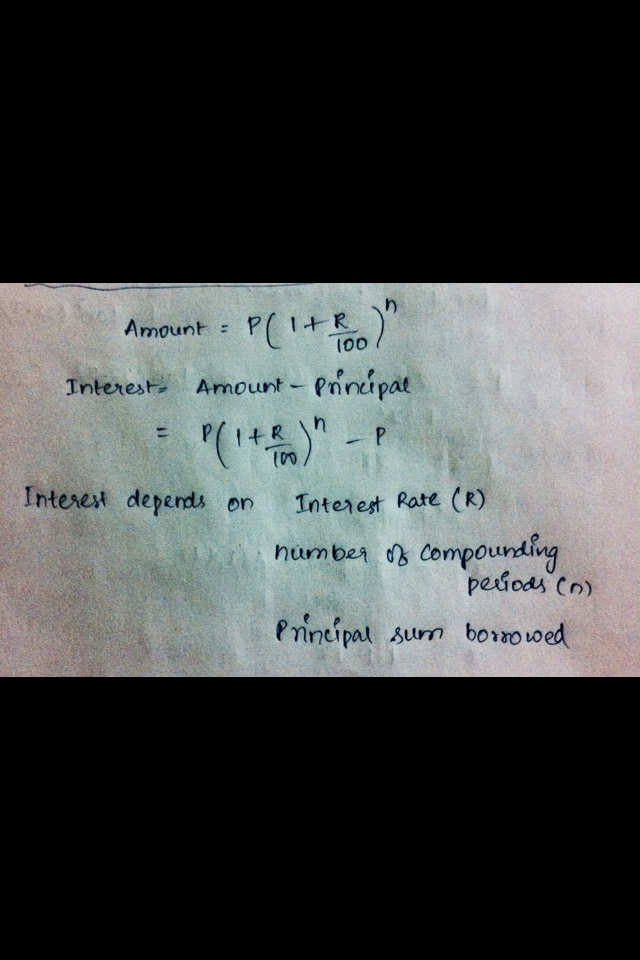# What factors determine the amount of interest earned on a fixed principal?

What factors determine the amount of interest earned on a fixed
principal​ (such as a​ lump-sum deposit into an​ account)? Select all that apply. A. Interest rate B. Number of compounding periods C. Frequency of periodic payments D. None of the above